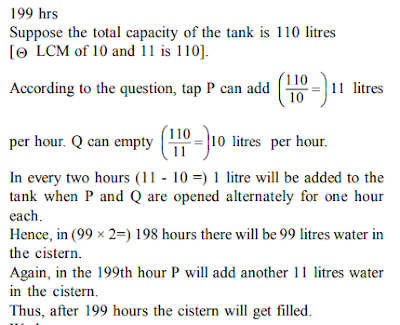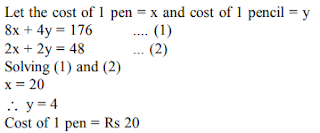# SBI Clerk 2018: Quantitative Aptitude Quiz for Prelims – 9

0
(0)

Hello and welcome to exampundit. Here is a set of Quantitative Aptitude Quiz for Prelims exam of SBI Clerk 2018.

1. In a partnership business A invests Rs 30,000 for three years, B invests Rs 40,000 for 2 1/2 years, C invests Rs 20,000 for two years and D invests Rs 10,000 for five years. Out of the total profit made, C gets a share of 16% as a ‘working partner’ before the profit is further distributed amongst all of them. What would be the share of C in the total profit of Rs 1,20,000?

1) Rs 32,400

2) Rs 36,000

3) Rs 36,300

4) Rs 28,800

5) Rs 33,600

Option: 5

Explanation:

1. A tap P can fill a tank in 10 hours. Another tap Q can empty the tank in 11 hours. The taps P and Q are opened already for one hour each. If P is opened first then after how many hours will the empty tank get filled?

1) 220 hours

2) 110 hours

3) 200 hours

4) 202 hours

5) None of these

Option: 5

Explanation:1. Six coins of equal weight, made of gold and silver mixed, were melted together and recast. In one, gold and silver were in the ratio 2 : 3; in two others, of 3 : 5; and in the rest of 5 : 4. In what ratio were the gold and silver be mixed in the new coins?

1) 169 : 191

2) 169 : 192

3) 169 : 197

4) 169 : 181

Option: 1

Explanation:1. A train leaves P for Q at the same time that a train leaves Q for P. The two trains meet at the end of 6 hours. The train from P to Q travelled 8 kilometers an hour more than the other. What would be the speed of the slower train if the distance from P to Q is 528 kilometers?

1) 40 km/hr

2) 30 km/hr

3) 48 km/hr

4) 50 km/hr

Option: 1

Explanation:1. The cost of 8 pens and 4 pencils is `176 and the cost of 2 pens and 2 pencils is `48. What is the cost of one pen?

1) `16

2) `14

3) `12

4) `18

5) None of these

Option: 5

Explanation:Directions (Q. 6-10): The following pie-charts show the percentage distribution of total votes of candidates in local election from 5 different villages during the year 1990 and 1991 and the table shows the ratio of general to reserved candidates among the selected candidates.1. How many reserved candidates were selected in 1990 in city C?

A) 1500

B) 1817

C) 2500

D) 2457

E) None

Option: B

Explanation: 0.23*25680*4/13=1817

1. In city E in 1990, the government decided to open a free insurance scheme for all the reserved candidates selected and hence distributed 879 forms for the same in the first slot. How many reserved candidates were kept pending for the 2nd slot ?

A) 65

B) 84

C) 74

D) 76

E) None

Option: B

Explanation:

0.1*25680*3/8=963

963-879=84

1. Due to sudden increase in the number of seats in the election in 1991 in D, 17% extra general candidates were selected. How many additional general candidates in D were selected ?

A) 750

B) 729

C) 547

D) 658

E) None

Option: B

Explanation:

0.28*32560*8/17=4290

0.17*4290=729

1. Maximum number of general candidates selected in 1990 is from which of the following villages?

A) E

B) B

C) D

D) A

E) C

Option: E

Explanation:

A=0.11*25680*6/11=1541

B=0.27*25680*7/15=3236

C=0.23*25680*9/13=4089

D=0.29*25680*1/4=1862

E=0.1*25680*5/8=1605

1. In 1991, village B’s panchayat declared that for all the reserved selected candidates , 3625 lakhs were allocated per candidate for development purposes. What is the total amount of money allocated by B’s panchayat?

A) 8,23,85,875 lakhs

B) 10,20,35,875 lakhs

C) 9,23,35,875 lakhs

D) 1,23,35,875 lakhs

E) 3,35,875 lakhs

Option: D

Explanation:

0.19*32560*11/20=3403

3625*3403=1,23,35,875 lakhs

Average rating 0 / 5. Vote count: 0

No votes so far! Be the first to rate this post.

We are sorry that this post was not useful for you!

Let us improve this post!

Tell us how we can improve this post?